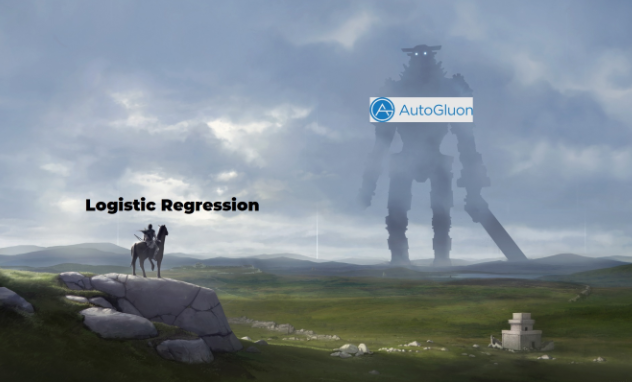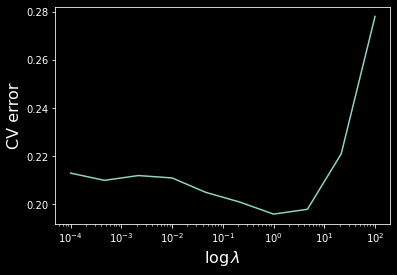Let’s compare logistic regression vs AutoGluon, an easy-to-implement AutoML library!We’ll use spambase data from UCI ML Repository which has $4601$ examples, $57$ features and $0/1$ (no-spam/spam) labels to find out which algorithm is better at detecting spam.

Snapshot of data

# $X_1$ $X_2$ $X_2$ $\cdots$ $X_{56}$ $X_{57}$ $Y$
0 $.00$ $.64$ $.64$ $\cdots$ $61$ $278$ $1$
1 $.21$ $.28$ $.50$ $\cdots$ $101$ $1028$ $1$
$\vdots$ $\vdots$ $\vdots$ $\vdots$ $\ddots$ $\vdots$ $\vdots$ $\vdots$
4600 $.00$ $.00$ $.65$ $\cdots$ $5$ $40$ $0$

To keep it simple, let’s ignore the data aspects (class imbalance, normalization, etc).

## Logistic Regression

We’ll implement a logistic regression with regularization from scratch (using numpy only).

### Import Spambase Data

First import the data, change encoding $0/1$ to $-1/1$ for convenience and do train-test split.

import numpy as np
np.random.seed(1)
+ "spambase/spambase.data", delimiter=',')
data = np.random.permutation(data)
data[:, -1] = (lambda x: x*2 - 1)(data[:, -1])  # switch to -1/1

train_y, test_y = data[: 3000, -1:], data[3000:, -1:]  # (3000, 1), (1601, 1)
train_x, test_x = data[: 3000, :-1], data[3000:, :-1]  # (3000, 57), (1601, 57)


Before we proceed, let’s do a very basic feature engineering step: add a column of $1$’s (intercept term) and an extra “indicator” feature that is $1$ if the corresponding $x$ is positive and $0$ otherwise, so that $[3,0,1]$ will turn to $[1, 3, 0, 1, 1, 0, 1]$.

def phi(x):
''' adds a column of ones
and all second-order combinations of features
'''
m = x.shape
x1 = np.hstack((np.ones((m, 1)), x))
x1 = np.hstack((x1, (x > 0).astype(float)))
return x1


### Loss & Optimizer

It helps to write down the loss, the gradient of the loss, and the Hessian of the loss for logistic regression. The $\ell_2$-penalized loss is ($m$ is sample size, $n$ is dimensionality)

\begin{align*} L &= \left[\sum_{i=1}^m \ln(1+e^{-y_i\mathrm{x_i}' \mathrm{w}})\right] + \frac{\lambda}{2}\sum_{j=2}^n w_j^2 \end{align*}.

Note that the first weight is not penalized since it corresponds to the intercept. The gradient and Hessian are

\begin{align*} \nabla_\mathrm{w} L &= - \left[\sum_{i=1}^m (1-s_i)y_i \mathrm{x}_i'\right] + \lambda\mathrm{w}^* \\ \nabla_\mathrm{w}^2 L &= \left[\sum_{i=1}^m s_i(1-s_i)\mathrm{x}_i\mathrm{x}_i'\right] + \lambda I^*_n \end{align*}

where

$s_i = (1+e^{-y_i\mathrm{x}'_i \mathrm{w}})^{-1},\qquad \mathrm{w}^* = \begin{bmatrix} 0 & w_2 & \cdots & w_n \end{bmatrix}', \qquad I^*_n = \begin{bmatrix} 0 & 0 & \cdots & 0 \\ 0 & 1 & \cdots & 0 \\ \vdots & \vdots & \ddots & \vdots \\ 0 & 0 & \cdots & 1 \end{bmatrix}$.

def lrloss(w, x, y, lmbda):
''' logistic loss '''
wstar = w.copy()
wstar = 0
return (np.sum(np.log(1+np.exp(-y*(x@w))), 0).T
+ .5*lmbda*np.sum(wstar*wstar))

s = 1/(1+np.exp(-y*(x@w)))
wstar = w.copy()
wstar = 0
return -np.sum((1-s)*x*y, 0)[:, np.newaxis] + lmbda*wstar

def lrhess(w, x, y, lmbda):
''' logistic hessian '''
s = 1/(1+np.exp(-y*(x@w)))
istar = np.eye(w.shape)
istar[0, 0] = 0
return x.T@np.diag((s*(1-s))[:, 0])@x + lmbda*istar


### Optimization

To estimate the weights, let’s implement a Newton-Raphson optimizer,

\begin{align*} \mathrm{w}_{t+1} = \mathrm{w}_t - H^{-1}_{\mathrm{w}_t} g_{\mathrm{w}_t} \end{align*},

where $H$ is the Hessian matrix and $g$ is the gradient vector.

This method is relatively straightforward, except when it doesn’t work.

We’ll check if the loss decreased after each iteration to alternate b/w gradient and Newton steps. Each time a second-order step does not decrease the loss, double the step size and try taking a gradient step. If it also fails, keep halving the step size until gradient descent succeeds (leads to smaller loss). As soon as it succeeds, return to second-order steps. Once the step sizes become too small, we are done.

(not a great implementation but demonstrates the idea)

def newton(w, fn, gradfn, hessfn):
''' newton-raphson optimizer'''
oldf = fn(w)
eta = 1
while True:
h = hessfn(w)
neww = w - np.linalg.solve(h, g)  # newton's step
newf = fn(neww)
if newf >= oldf:                  # failed to decrease the loss :(
eta *= 2                      # double the step size
while eta > 1e-10:
neww = w - eta*g          # gradient step
newf = fn(neww)
if newf < oldf:           # exit if loss decreased!
break
eta *= .5                 # o/w keep halving
if eta < 1e-10:               # finish if step size too small
return w
oldf = newf
w = neww


### Train time!

We should be able to train the model now!

def trainlr(x, y, lmbda):
''' training to produce w estimate '''
w0 = np.zeros((x.shape, 1))  # initiating at zero works well for LR
return newton(w0, lambda w: lrloss(w, x, y, lmbda),
lambda w: lrgrad(w, x, y, lmbda),
lambda w: lrhess(w, x, y, lmbda))

def lrerrorrate(x, y, w):
''' share of incorrectly classified '''
return np.sum(y*x@w < 0)/y.shape

lmb = .1
mytrain_x, mytest_x = phi(train_x), phi(test_x)
myw = trainlr(mytrain_x, train_y, lmb)
print(lrerrorrate(mytest_x, test_y, myw))


Which gives a training accuracy of about $5.2\%$ !### Cross-Validation

Let’s implement 3-fold cross-validation and plot the cv error as a function of lambda.

def xvalideval(x, y, lmbda):
''' validation error '''
nfold = 3
px = phi(x)
m = x.shape
splits = np.linspace(0, m, nfold+1).astype(int)
v = 0
for low, high in zip(splits[0:-1], splits[1:]):
# validation bucket, from low to high
validx = px[low:high, :]
validy = y[low:high, :]
# training bucket, everything else
trainx = np.vstack((px[:low, :], px[high:, :]))
trainy = np.vstack((y[:low, :], y[high:, :]))

v += lrerrorrate(validx, validy, trainlr(trainx, trainy, lmbda))
return v

ls = np.logspace(-4, 2, 10)
xverr = ls.copy()
bestv, bestl = None, None
for i, l in enumerate(ls):
xverr[i] = xvalideval(train_x, train_y, l)
if bestv is None or bestv > xverr[i]:
bestv = xverr[i]
bestl = l

import matplotlib.pyplot as plt
plt.semilogx(ls, xverr)
plt.ylabel('CV error', size=16)
plt.xlabel('$\log \lambda$', size=16)
plt.show()Hence we have $\lambda \approx 1$ achieving the lowest validation error.

testerr = lrerrorrate(phi(test_x), test_y, trainlr(phi(train_x), train_y, bestl))
print(f"lambda = {bestl}, test error = {testerr}")


Producing the test error of $\boxed{5.1\%}$, not bad!

## AutoGluon

For comparison, let’s see the performance of an AutoML library which will automatically process the data, train and combine a range of models.

from autogluon.tabular import TabularPredictor, TabularDataset
train_data, test_data = TabularDataset(data[:3000]), TabularDataset(data[3000:])
predictor = TabularPredictor(label=57).fit(train_data)
y_pred = predictor.predict(test_data.iloc[:, :57])
testerr = np.sum(test_y.transpose()*y_pred.to_numpy() < 0)/test_y.shape
print(f"test error = {testerr}")


Yielding test error of $\boxed{3.6\%}$ , beating LR by $1.5\%$ !

## Conclusion